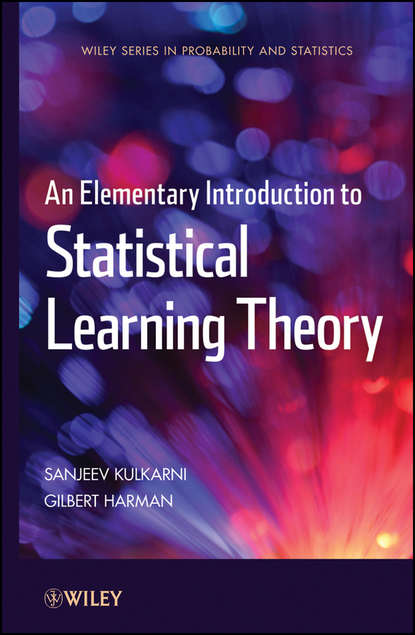An Elementary Introduction to Statistical Learning Theory

# An Elementary Introduction to Statistical Learning Theory

Автор
Год написания книги
2018
A thought-provoking look at statistical learning theory and its role in understanding human learning and inductive reasoning A joint endeavor from leading researchers in the fields of philosophy and electrical engineering, An Elementary Introduction to Statistical Learning Theory is a comprehensive and accessible primer on the rapidly evolving fields of statistical pattern recognition and statistical learning theory. Explaining these areas at a level and in a way that is not often found in other books on the topic, the authors present the basic theory behind contemporary machine learning and uniquely utilize its foundations as a framework for philosophical thinking about inductive inference. Promoting the fundamental goal of statistical learning, knowing what is achievable and what is not, this book demonstrates the value of a systematic methodology when used along with the needed techniques for evaluating the performance of a learning system. First, an introduction to machine learning is presented that includes brief discussions of applications such as image recognition, speech recognition, medical diagnostics, and statistical arbitrage. To enhance accessibility, two chapters on relevant aspects of probability theory are provided. Subsequent chapters feature coverage of topics such as the pattern recognition problem, optimal Bayes decision rule, the nearest neighbor rule, kernel rules, neural networks, support vector machines, and boosting. Appendices throughout the book explore the relationship between the discussed material and related topics from mathematics, philosophy, psychology, and statistics, drawing insightful connections between problems in these areas and statistical learning theory. All chapters conclude with a summary section, a set of practice questions, and a reference sections that supplies historical notes and additional resources for further study. An Elementary Introduction to Statistical Learning Theory is an excellent book for courses on statistical learning theory, pattern recognition, and machine learning at the upper-undergraduate and graduate levels. It also serves as an introductory reference for researchers and practitioners in the fields of engineering, computer science, philosophy, and cognitive science that would like to further their knowledge of the topic.

## Читать онлайн

Авторизуйтесь чтобы можно было оставлять комментарии

## Отзывы

список сообщений пуст